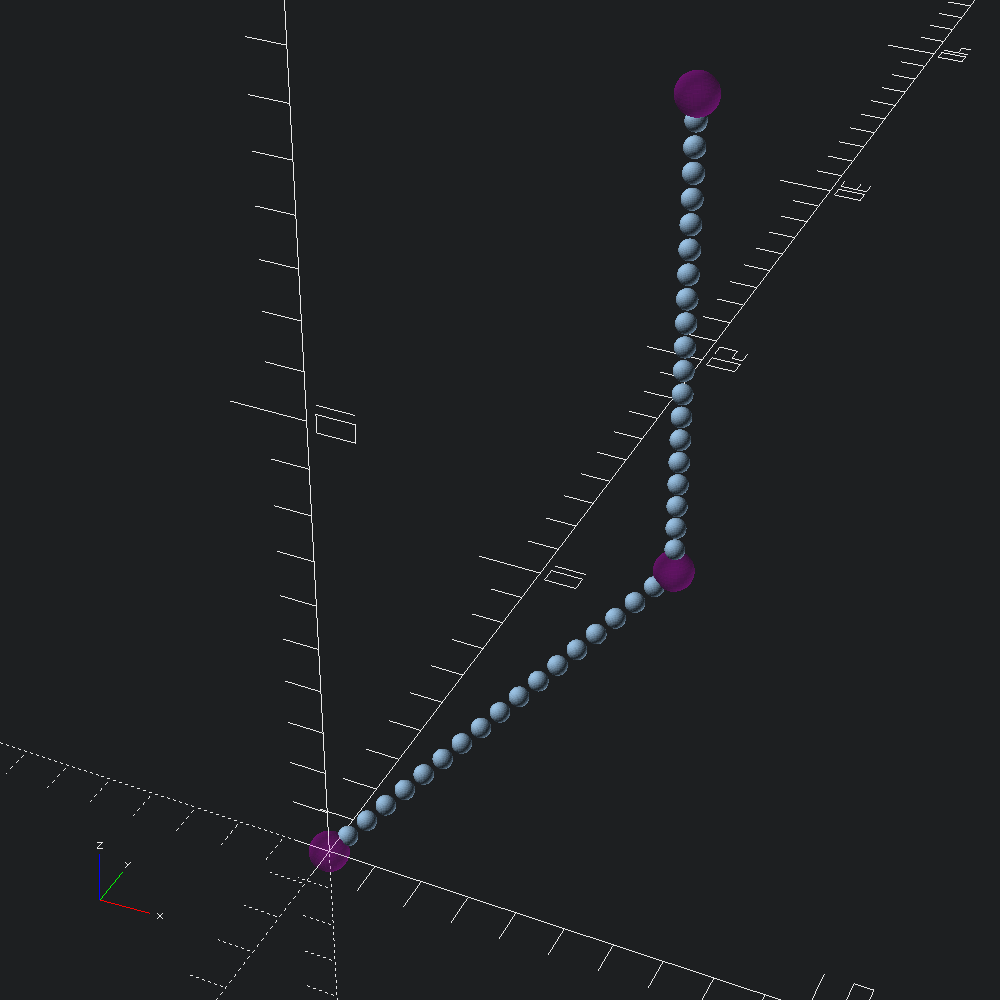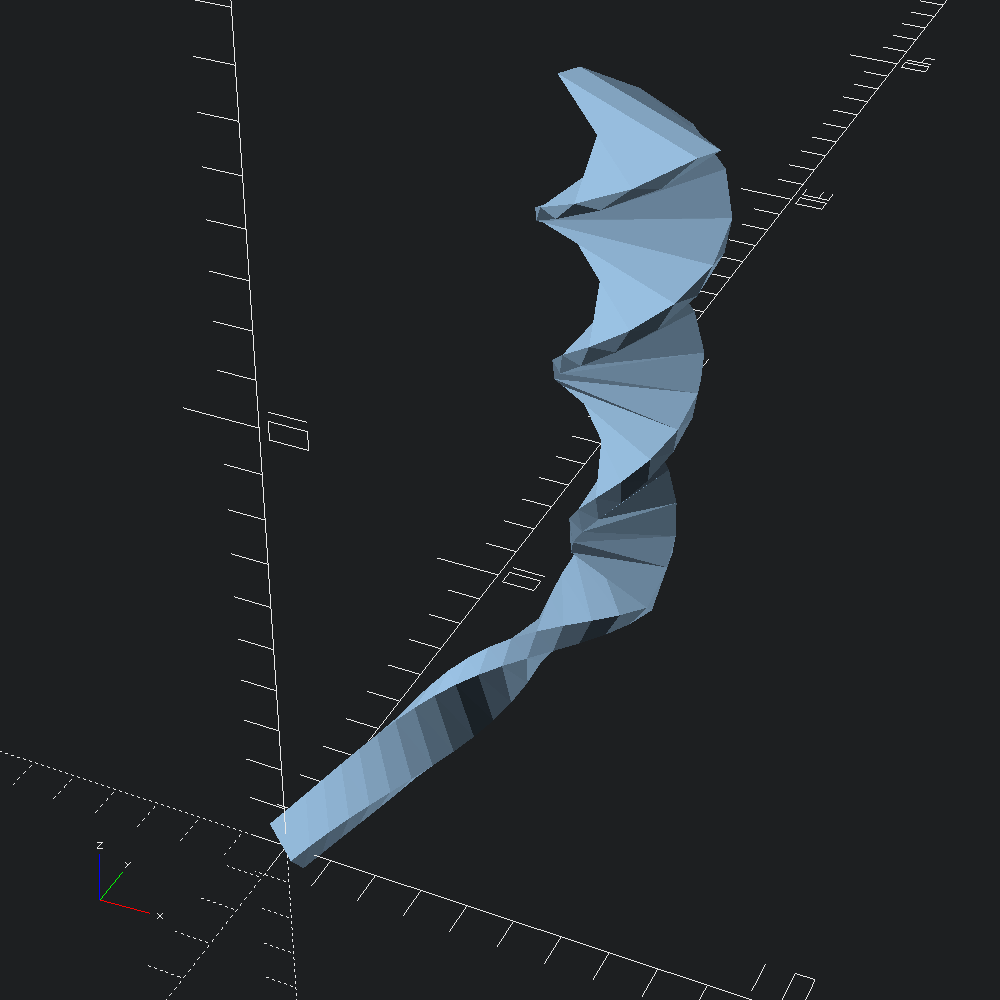# Path resampling and scaling/twisting sweeps

``````open OCADml

Oftentimes, we would just like to define our paths without colinear points, such that it only consists of corners. However, some applications call for more fine sampling, and in those times we can reach for `Path3.resample` or `Path3.subdivide`. For this example, the exact number of points isn't a concern, so we'll specify a new point spacing as our `~freq`.

``````let path = [ v3 0. 0. 0.; v3 5. 5. 5.; v3 5. 5. 15. ]
let resampled = Path3.subdivide ~freq:(`Spacing 0.5) path``````

Lets visualize our original `path`, and the `resampled` points with `Debug.show_path3` to get a sense of how fine our `~freq` parameter has gotten us.

``````let () =
let old_marks =
let f _ = Scad.(color ~alpha:0.2 Color.Magenta @@ sphere ~fn:36 0.4) in
Debug.show_path3 f path
and new_marks = Debug.show_path3 (fun _ -> Scad.sphere ~fn:36 0.2) resampled inOne such alluded to application for resampling, is when additional transformations such as scaling and twisting along a path extrusion is desired.

``````let () =
let mesh =
Mesh.path_extrude
~scale_ez:(v2 0.42 0., v2 1. 1.)
~twist_ez:(v2 0.42 0., v2 1. 1.)
~scale:(v2 4. 1.)
~twist:(Float.pi *. 4.)
~path:resampled
@@ Poly2.square ~center:true (v2 1. 0.5)
in